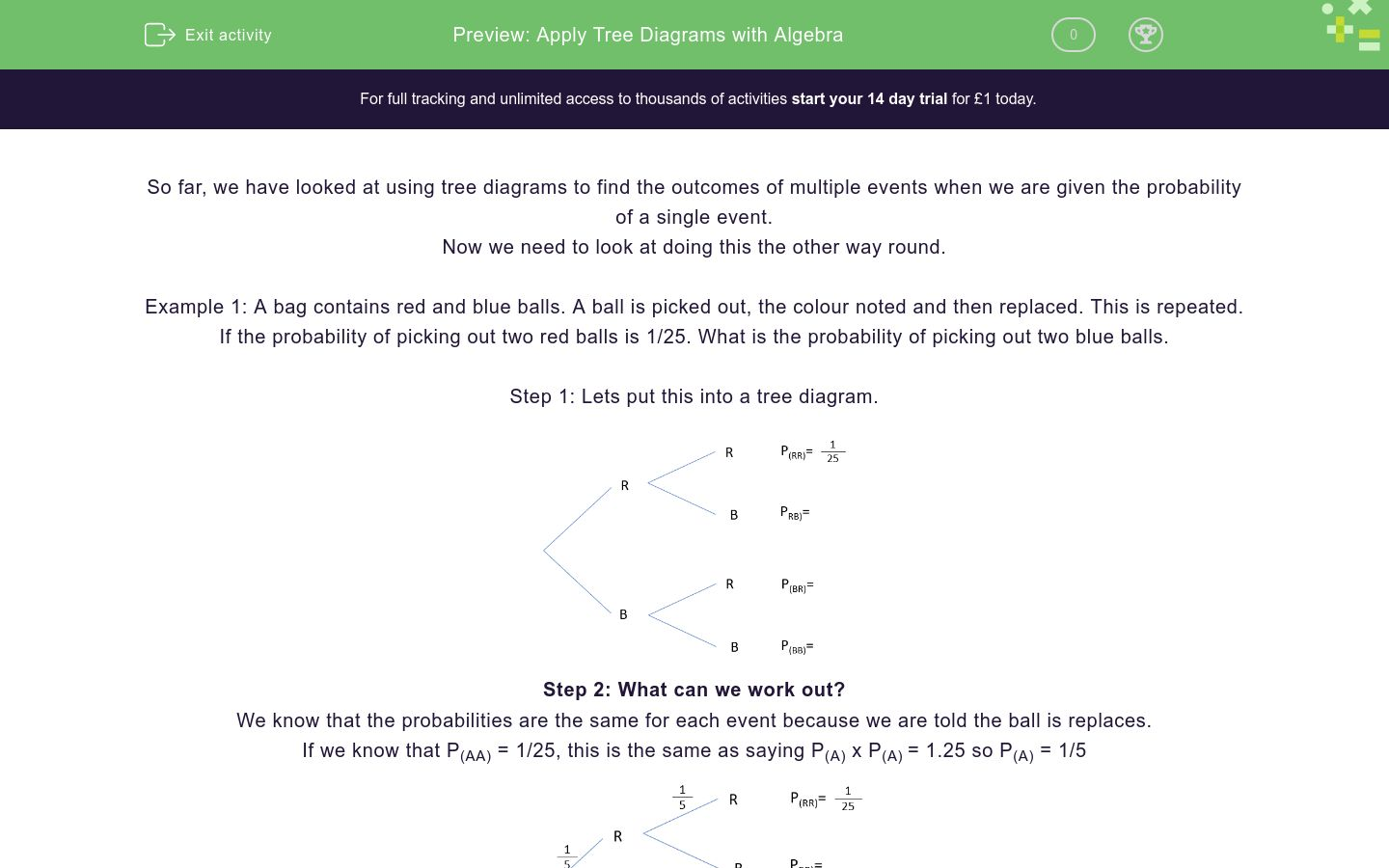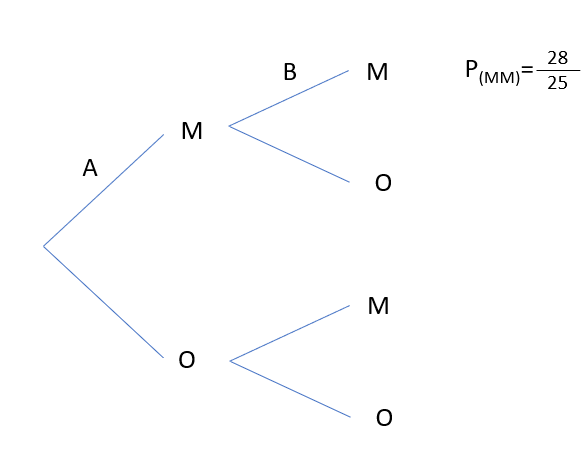# Apply Tree Diagrams with Algebra

In this worksheet, students will apply algebra to tree diagrams.Key stage:  KS 4

GCSE Subjects:   Maths

GCSE Boards:   AQA, Eduqas, Pearson Edexcel, OCR

Curriculum topic:   Probability

Curriculum subtopic:   Probability, Combined Events and Probability Diagrams

Difficulty level:### QUESTION 1 of 10

So far, we have looked at using tree diagrams to find the outcomes of multiple events when we are given the probability of a single event.

Now we need to look at doing this the other way round.

Example 1: A bag contains red and blue balls. A ball is picked out, the colour noted and then replaced. This is repeated.

If the probability of picking out two red balls is 1/25. What is the probability of picking out two blue balls.

Step 1: Lets put this into a tree diagram.Step 2: What can we work out?

We know that the probabilities are the same for each event because we are told the ball is replaces.

If we know that P(AA) = 1/25, this is the same as saying P(A) x P(A) = 1.25 so P(A) = 1/5Step 3: Work out the other event.

We know that A and B are exhaustive, so P(B) must equal 4/5Step 4: Complete the rest of the tree diagram.Step 5: Use this to answer the question.

We were intially asked for the Probability of 2 blue balls. From the tree diagram, we can now see that this is 16/25

Complete the following sentence.

For this question, draw a tree diagram before you answer the question.

A bag contains only Black and White balls.

A ball is withdrawn and the colour noted. It is replaced and another is taken out.

If the probability of getting 2 blue balls is 9/49, find the probability of getting 2 reds.

For this question, draw a tree diagram before you answer the question.

A bag contains only Black and White balls.

A ball is withdrawn and the colour noted. It is replaced and another is taken out.

If the probability of getting 2 blue balls is 9/49, find the probability I will get exactly 1 red ball

12/49

24/49

For this question, draw a tree diagram before you answer the question.

A bag contains only Black and White balls.

A ball is withdrawn and the colour noted. It is replaced and another is taken out.

If the probability of getting 2 blue balls is 9/49, find the probability I will get at least one red

A bag contains 10 sweets. Some are mint and some are orange.

I take two sweets.

For the tree diagram shown, which of these options are the correct algebraic terms for A and B?A =
 x 10
And B =
 x 9

A =
 x 10
And B =
 x-1 9

A bag contains 10 sweets. Some are mint and some are orange.

I take two sweets.

For the tree diagram shown, which of these options are the correct algebraic terms for A and B?We have just worked out the two individual probabilities for Mint as x/10 and x-1 /9

Use this to find out the number of mint sweets in the bag.

A =
 x 10
And B =
 x 9

A =
 x 10
And B =
 x-1 9

A bag contains 10 sweets. Some are mint and some are orange.

I take two sweets.

We have now found that there are 8 mint sweets.

What is the probability I don't get a mint sweet?

I bag contains red and blue balls.

If P(Red) = a,

I bag contains red and blue balls.

If P(Red) = a

If I take out two balls, match the probabilities with their algebraic forms.

## Column B

P(RR)
a(1-a)
P(RB)
a2
P(BR)
1 - a2
P(BB)
a(1-a)

I bag contains red and blue balls.

If P(Red) = a

If I remove two balls (with replacement)

Find the probability exactly one of them will be blue.

Give your answer in its simple factorised form. Don't put any spaces between characters.

• Question 1

Complete the following sentence.

EDDIE SAYS
It's all about doing it backwards. If you are given the outcomes, you can work backwards using algebra and then work out the rest of the table from the start.
• Question 2

For this question, draw a tree diagram before you answer the question.

A bag contains only Black and White balls.

A ball is withdrawn and the colour noted. It is replaced and another is taken out.

If the probability of getting 2 blue balls is 9/49, find the probability of getting 2 reds.

16/49
EDDIE SAYS
We know that P(BB) = 9/49 This means that P(B) = 3/7 From this, we can find that P(R) = 4/7 All we have to do now is find the probability of RR
• Question 3

For this question, draw a tree diagram before you answer the question.

A bag contains only Black and White balls.

A ball is withdrawn and the colour noted. It is replaced and another is taken out.

If the probability of getting 2 blue balls is 9/49, find the probability I will get exactly 1 red ball

24/49
EDDIE SAYS
We know that P(BB) = 9/49 This means that P(B) = 3/7 From this, we can find that P(R) = 4/7 There are two ways I can get exactly one red. RB and BR We need to find these two probabilities and then add them
• Question 4

For this question, draw a tree diagram before you answer the question.

A bag contains only Black and White balls.

A ball is withdrawn and the colour noted. It is replaced and another is taken out.

If the probability of getting 2 blue balls is 9/49, find the probability I will get at least one red

40/49
EDDIE SAYS
We know that P(BB) = 9/49 This means that P(B) = 3/7 From this, we can find that P(R) = 4/7 There are three ways I can get at least 1 red RB, BR and RR We need to find these three probabilities and then add them. You could also use the fact that P(at least) = 1 - P(none) P(at least 1 red) = 1 - P (BB) It'll give the same answer and be a bit quicker.
• Question 5

A bag contains 10 sweets. Some are mint and some are orange.

I take two sweets.

For the tree diagram shown, which of these options are the correct algebraic terms for A and B?A =
 x 10
And B =
 x-1 9
EDDIE SAYS
We know that this is a conditional probability question so we have to reduce the numbers in the second mint by 1. Because we don't know how many mints there are, we have to call this X and the second mint will have 1 less so it will be x-1
• Question 6

A bag contains 10 sweets. Some are mint and some are orange.

I take two sweets.

For the tree diagram shown, which of these options are the correct algebraic terms for A and B?We have just worked out the two individual probabilities for Mint as x/10 and x-1 /9

Use this to find out the number of mint sweets in the bag.

EDDIE SAYS
We can see that AxB = 28/45 We can use this to create a quadratic formula.. (x/10) x (x-1/9) = 28/45 Rearranging this gives... x2
• Question 7

A bag contains 10 sweets. Some are mint and some are orange.

I take two sweets.

We have now found that there are 8 mint sweets.

What is the probability I don't get a mint sweet?

1/25
EDDIE SAYS
For this, we're now looking for P(OO) The probability for the first sweet being orange is 2/10 The probability the second is orange if the first is orange is 1/9 Multiply, cancel and answer...
• Question 8

I bag contains red and blue balls.

If P(Red) = a,

1-a
1 - a
1- a
1 -a
EDDIE SAYS
We have to work on the fact here that the bag only contains red and blue. This means the probabilities of red and blue MUST add to 1 P(red) + P(blue) = 1 P (Blue) = 1 - P(red)
• Question 9

I bag contains red and blue balls.

If P(Red) = a

If I take out two balls, match the probabilities with their algebraic forms.

## Column B

P(RR)
a2
P(RB)
a(1-a)
P(BR)
a(1-a)
P(BB)
1 - a2
EDDIE SAYS
This all relies on the previous question P(R) = a P(B) = 1-a The rest of the compound probabilities can be found by multiplying these together in the correct way.
• Question 10

I bag contains red and blue balls.

If P(Red) = a

If I remove two balls (with replacement)

Find the probability exactly one of them will be blue.

Give your answer in its simple factorised form. Don't put any spaces between characters.

2a(1-a)
EDDIE SAYS
There are two ways this can happen RB which has a probability of a(1-a) BR which has a probability of 1(1-a) Adding these together gives...
---- OR ----

Sign up for a £1 trial so you can track and measure your child's progress on this activity.

### What is EdPlace?

We're your National Curriculum aligned online education content provider helping each child succeed in English, maths and science from year 1 to GCSE. With an EdPlace account you’ll be able to track and measure progress, helping each child achieve their best. We build confidence and attainment by personalising each child’s learning at a level that suits them.

Get started